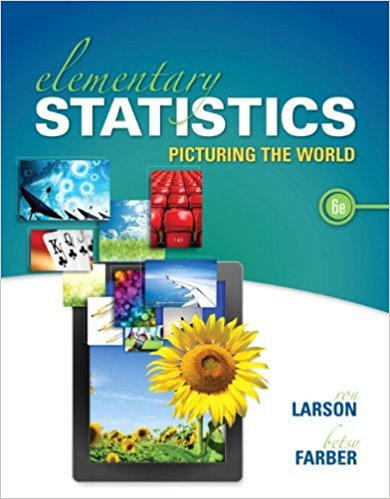×
Get Full Access to Elementary Statistics: Picturing The World - 6 Edition - Chapter 3.2 - Problem 27e
Get Full Access to Elementary Statistics: Picturing The World - 6 Edition - Chapter 3.2 - Problem 27e

×

# Blood Types The probability that a person in the UnitedISBN: 9780321911216 66

## Solution for problem 27E Chapter 3.2

Elementary Statistics: Picturing the World | 6th Edition

• Textbook Solutions
• 2901 Step-by-step solutions solved by professors and subject experts
• Get 24/7 help from StudySoup virtual teaching assistantsElementary Statistics: Picturing the World | 6th Edition

4 5 1 290 Reviews
25
5
Problem 27E

Problem 27E

Blood Types The probability that a person in the United States has type B+ blood is 9%. Five unrelated people in the United States are selected at random. (Source: American Association of Blood Banks)

(a) Find the probability that all five have type B+ blood.

(b) Find the probability that none of the five have type B+ blood.

(c) Find the probability that at least one of the five has type B+ blood.

(d) Which of the events can be considered unusual? Explain.

Step-by-Step Solution:

Step 1 of 4

(a)

The probability that a person in the U.S. has type B+ blood is 9%.

Five unrelated people in the U.S. are selected at random.

We are asked to find the probability that all five have type B+ blood.

Using independence rule of probability, we can write the probability that all five have type B+ blood,[since the probability that a person in the U.S. has type B+ blood is 9% or 0.09]

Hence the probability isStep 2 of 4

Step 3 of 4

##### ISBN: 9780321911216

Unlock Textbook Solution